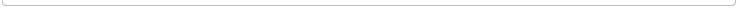﻿南方科技大学杨将副教授应邀来我院做学术报告-湖南师范大学数学与统计学院首页 | 学院概况 | 师资队伍 | 本科生教育 | 研究生教育 | 学科建设 | 科学研究 | 党政工作 | 资料下载

 学术报告学术报告
 当前位置： 首页>>学术报告>>正文南方科技大学杨将副教授应邀来我院做学术报告 2019-06-20 15:29     (访问量：) 学术报告    报告题目：Time-Fractional Allen-CahnEquations: Analysis and Numerical Methods     报告人：杨将  （南方科技大学副教授）     报告时间：6月22日下午16:30—17:15     报告地点：数统院307                                                数学与统计学院                                                   2019.6.20     报告摘要：In this work, weconsider a time-fractional Allen-Cahn equation, where the conventional firstorder time derivative is replaced by a Caputo fractional derivative with order$\alpha\in(0,1)$. First, the well-posedness and (limited) smoothing propertyare systematically analyzed, by using the maximal $L^p$ regularity offractional evolution equations and the fractional Gr\"onwall's inequality.We also show the maximum principle like their conventional local-in-timecounterpart. Precisely, the time-fractional equation preserves the propertythat the solution only takes value between the wells of the double-wellpotential when the initial data does the same. Second, after discretizing thefractional derivative by backward Euler convolution quadrature, we developseveral unconditionally solvable and stable time stepping schemes, i.e., convexsplitting scheme, weighted convex splitting scheme and linear weighted stabilizedscheme. Meanwhile, we study the discrete energy dissipation property (in aweighted average sense), which is important for gradient flow type models, forthe two weighted schemes. Finally, by using a discrete version of fractionalGr\"onwall's inequality and maximal $\ell^p$ regularity, we prove that theconvergence rates of those time-stepping schemes are $O(\tau^\alpha)$ withoutany extra regularity assumption on the solution. We also present extensivenumerical results to support our theoretical findings and to offer new insighton the time-fractional Allen-Cahn dynamics. 【关闭窗口】Copyright 2004-2019@ School of Mathematics and Statistics 电话: 0731-88872515   湖南师范大学数学与统计学院